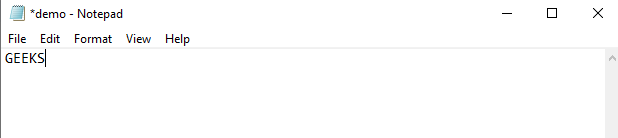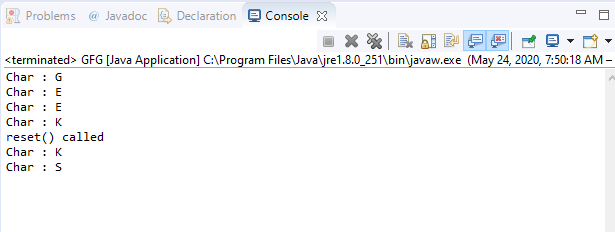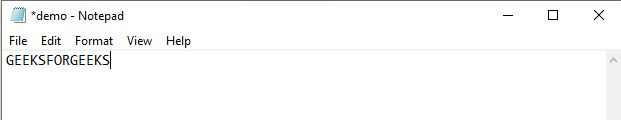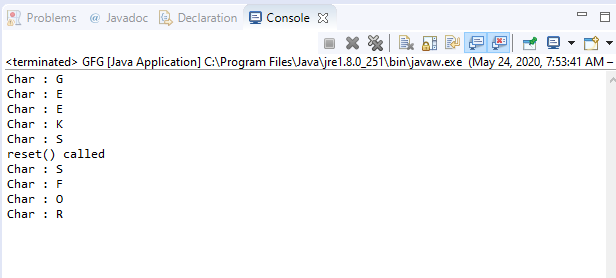GFG App
Open AppBrowser
Continue

# BufferedInputStream reset() method in Java with Examples

The reset() method of BufferedInputStream class in Java is used to reset the position of the stream to the position at the time the mark method was last called. It is used with the combination of mark() method of the same class.

General Contract:
There are two cases:

1. If mark() and reset() are supported i.e., if markSupported returns true,
• An IOException might be thrown if the mark() is not called since the creation of the input stream or the bytes that are read from the input stream since the last call of mark() method is greater than the argument to mark method at the last call.
• In case an IOException is not thrown, then the input stream is reset to a state such that all the bytes that are read after the most recent call of mark() is supplied again to subsequent callers of the read(). This is followed by the bytes that would have at reset() call.
2. If mark() and reset() are not supported i.e., if markSupported returns false,
• Calling reset() method might throw an IOException.
• In case an IOException is not thrown, then input stream is reset to a fixed state that depends on the particular type of the input stream. The supplied bytes to subsequent callers of the read() depend on the particular type of the input stream.

Syntax:

`public void reset()`

Overrides: The method overrides the reset class in FilterInputStream.

Parameters: This method does not accept any parameter.

Return value: This method does not return any value.

Exception: This method throws IOException if this stream has not been marked or mark has been invalidated or input stream has been closed by invoking the close() method or an I/O error occurs.

Below programs illustrates reset() method in BufferedInputStream class in IO package:

Program 1: Assume the existence of file “c:/demo.txt”.

 `// Java program to illustrate ` `// BufferedInputStream reset() method ` `import` `java.io.*; ` `public` `class` `GFG { ` `    ``public` `static` `void` `main(String[] args) ` `    ``{ ` ` `  `        ``// Create input stream 'demo.txt' ` `        ``// for reading containing text "GEEKS" ` `        ``FileInputStream inputStream ` `            ``= ``new` `FileInputStream( ` `                ``"c:/demo.txt"``); ` ` `  `        ``// Convert inputStream to ` `        ``// bufferedInputStream ` `        ``BufferedInputStream buffInputStr ` `            ``= ``new` `BufferedInputStream( ` `                ``inputStream); ` ` `  `        ``// Read and print characters one by one ` `        ``System.out.println( ` `            ``"Char : "` `            ``+ (``char``)buffInputStr.read()); ` `        ``System.out.println( ` `            ``"Char : "` `            ``+ (``char``)buffInputStr.read()); ` `        ``System.out.println( ` `            ``"Char : "` `            ``+ (``char``)buffInputStr.read()); ` ` `  `        ``// Mark is set on the input stream ` `        ``buffInputStr.mark(``0``); ` ` `  `        ``System.out.println( ` `            ``"Char : "` `            ``+ (``char``)buffInputStr.read()); ` ` `  `        ``System.out.println( ` `            ``"reset() called"``); ` ` `  `        ``// Reset() is invoked ` `        ``buffInputStr.reset(); ` ` `  `        ``// Read and print characters ` `        ``System.out.println( ` `            ``"Char : "` `            ``+ (``char``)buffInputStr.read()); ` `        ``System.out.println( ` `            ``"Char : "` `            ``+ (``char``)buffInputStr.read()); ` `    ``} ` `} `

Input:Output:Program 2: Assume the existence of file “c:/demo.txt”.

 `// Java program to illustrate ` `// BufferedInputStream reset() method ` `import` `java.io.*; ` `public` `class` `GFG { ` `    ``public` `static` `void` `main(String[] args) ` `    ``{ ` ` `  `        ``// Create input stream 'demo.txt' ` `        ``// for reading containing text ` `        ``// "GEEKSFORGEEKS" ` `        ``FileInputStream inputStream ` `            ``= ``new` `FileInputStream( ` `                ``"c:/demo.txt"``); ` ` `  `        ``// Convert inputStream to ` `        ``// bufferedInputStream ` `        ``BufferedInputStream buffInputStr ` `            ``= ``new` `BufferedInputStream( ` `                ``inputStream); ` ` `  `        ``// Read and print characters one by one ` `        ``System.out.println( ` `            ``"Char : "` `            ``+ (``char``)buffInputStr.read()); ` `        ``System.out.println( ` `            ``"Char : "` `            ``+ (``char``)buffInputStr.read()); ` `        ``System.out.println( ` `            ``"Char : "` `            ``+ (``char``)buffInputStr.read()); ` `        ``System.out.println( ` `            ``"Char : "` `            ``+ (``char``)buffInputStr.read()); ` ` `  `        ``// Mark is set on the input stream ` `        ``buffInputStr.mark(``0``); ` ` `  `        ``System.out.println( ` `            ``"Char : "` `            ``+ (``char``)buffInputStr.read()); ` ` `  `        ``System.out.println( ` `            ``"reset() called"``); ` ` `  `        ``// Reset() is invoked ` `        ``buffInputStr.reset(); ` ` `  `        ``// Read and print characters ` `        ``System.out.println( ` `            ``"Char : "` `            ``+ (``char``)buffInputStr.read()); ` `        ``System.out.println( ` `            ``"Char : "` `            ``+ (``char``)buffInputStr.read()); ` `        ``System.out.println( ` `            ``"Char : "` `            ``+ (``char``)buffInputStr.read()); ` `        ``System.out.println( ` `            ``"Char : "` `            ``+ (``char``)buffInputStr.read()); ` `    ``} ` `} `

Input:Output:My Personal Notes arrow_drop_up